# Acutance and SQF (Subjective Quality Factor)

View legacy documentation

Measurement of perceptual image sharpness

## Introduction to Acutance and SQF

Acutance and are measures of perceived print or display sharpness. SQF was used for years in the photographic industry but has remained unfamiliar to most photographers. Acutance is a relatively new measurement from the IEEE Camera Phone Image Quality (CPIQ) group. Both include the effects of

• MTF: the imaging system’s Modulation Transfer Function, which is synonymous with Spatial Frequency Response (SFR),
• CSF: the human eye’s Contrast Sensitivity Function (closely related to its MTF response, but including a low frequency rolloff),
• image display height, and
• viewing distance.

SQF was introduced in the paper, “An optical merit function (SQF), which correlates with subjective image judgments ,” by E. M. (Ed) Granger and K. N. Cupery of Eastman Kodak, published in Photographic Science and Engineering, Vol. 16, no. 3, May-June 1973, pp. 221-230. (If you do an Internet search, note that Granger’s name is often misspelled Grainger.) It was used by Kodak and Polaroid for product development and is used by Popular Photography for lens tests. This technical paper verified its correlation with viewer preference. But SQF is rarely mentioned on photography websites with the notable exception of Bob Atkinsexcellent description, which includes an explanation of Pop Photo’s methods.

Acutance is a perceptual measurement that is closely related to SQF, but differs in the details of the equation. It is defined in the IEEE CPIQ (Camera Phone Image Quality) Phase 2 specification, but this document gives little indication of how it should be displayed. Imatest displays it in the same way as SQF. Because of the CPIQ standardization work, which includes correlating Acutance numbers to perceptual quality loss (Just Noticeable Differences = JNDs) we generally recommend Acutance over SQF.

Acutance and SQF can be measured in many of Imatest’s sharpness modules: SFR, SFRplus, Star, Random/Dead Leaves, SFRreg, Checkerboard, and eSFR ISO.

## Acutance (or SQF) and MTF

The following table compares MTF and acutance (or SQF). In essence, MTF is a measurement of device or system sharpness; acutance and SQF are measurements of perceptual print or diaplay sharpness, derived from MTF, the contrast sensitivity function (CSF) of the human visual system, and viewing angle (based on an assumption about the relationship between print height and viewing distance).

 MTF (Modulation Transfer Function) Acutance or SQF (Subjective Quality Factor) Measures image contrast as a function of spatial frequency. Describes device or system sharpness. Indirectly related to perceived image sharpness in a print or display. Measures perceived sharpness as a function of print or display height and viewing distance. Describes viewer experience. Requires an assumption about viewing distance (can be constant or proportional to the square root or cube root of print height). Omits viewing distance and the human visual system. Includes the effects of viewing distance and the human visual system. Abstract: Plots of MTF (contrast) vs. spatial frequency require technical skill to interpret. Concrete: Plots of SQF (perceived sharpness) vs. print height require little interpretation. SQF has the same subjective meaning regardless of print size. It can be used to precisely answer questions like, “How much larger can I print with a 12.8 Megapixel full-frame DSLR than with an 8.3 Megapixel APS-C DSLR?” 1.0 at low spatial frequencies. Decreases at high spatial frequencies, but may peak at intermediate frequencies due to sharpening. A peak over 1.4 indicates oversharpening, which can result un unpleasant “halos” at edges, especially for large print sizes. 100 (%) for small print heights. Decreases for large print heights, but may increase at intermediate print heights due to sharpening. A peak value over about 105% may indicate oversharpening (and over 108% definitely indicates oversharpening). In such cases you should examine the MTF plot and edge profile. The frequency where it drops by half (MTF50) is a reasonable indicator of relative perceived sharpness, but requires interpretation and is not as precise as SQF. An SQF difference of 5 corresponds to a perceptible change in in sharpness— somewhat more than one “Just Noticeable Difference” (JND). SQF can be used as the basis of a ranking system. JND for Acutance is displayed by Imatest. It is described below. Familiar to imaging scientists Gradually becoming familiar to a wider public, though vanishing resolution is still better known. SQF is unfamiliar in the imaging industry. It was used internally in Kodak and Polaroid, but difficult to measure prior to the advent of digital imaging. Acutance is a relatively new measurement that is becoming familiar thanks to the IEEE CPIQ group. All the measurements (MTF, SQF, and acutance) are strongly affected by sharpening, which is routinely performed to improve perceived sharpness, hence SQF would be expected to increase.

Acutance and SQF are calculated as a part of the SFR, SFRplus, Star, Random/Dead Leaves, SFRreg, Checkerboard, and eSFR ISO modules. To display it, check the Acutance/SQF checkbox in the settings box, making sure the Acutance/SQF settings (described below) are appropriate. Here is a sample result.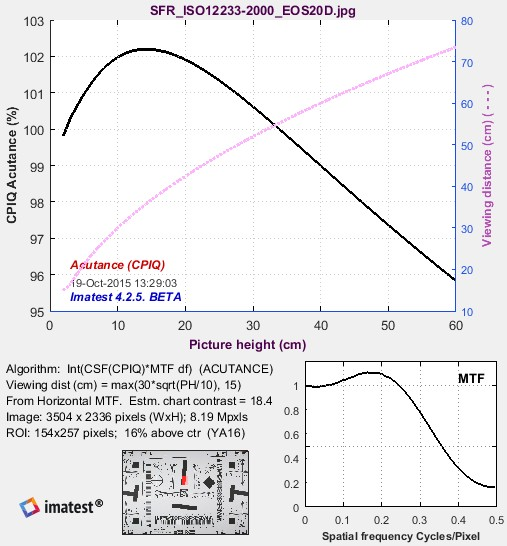Acutance, based on the assumption that viewing distance = square root of image height.

The solid black line in the top plot shows acutance for print heights from 2 to 60 cm (~2 to 24 inches), assuming viewing distance (cm) = 30 √(picture height/10), which is in the ballpark for gallery viewing conditions. Acutance goes over 100 because the image has a modest MTF peak from sharpening. The top plot also shows viewing distance ( – – – ). The plot on the lower right is the MTF, which is displayed in the main SFR/MTF figure, but repeated here to make this figure self-contained. The lower middle contains a thumbnail of the image showing the selected ROI in red. The text on the left contains calculation details and image properties.

## What do the acutance and SQF numbers mean?

SQF— Ed Granger developed SQF to be linearly proportional to perceived sharpness. A change in SQF of 5 corresponds to a perceptible change in sharpness— somewhat more than one “Just Noticeable Difference” (JND). Since we do not have our own database of SQF impressions we’ll draw on the experience of others.

Popular Photography has been using SQF for testing lenses for years. They’ve developed the only generally-available SQF ranking system. Their scale isn’t quite linear. {C, C+} takes up 20 SQF units; twice as many as {A, A+}, {B, B+}, or D. But it seems to be a good starting point for interpreting the numbers.

 A+ A B+ B C+ C D F 94-100 89-94 84-89 79-84 69-79 59-69 49-59 Under 49

We encourage readers to examine Popular Photography‘s lens test results and to search its site for SQF. Imatest results should correlate with Pop Photo‘s results, but there are a few significant differences.

• Pop Photo measures SQF for lenses alone, while Imatest measures SQF for the entire imaging system. This means that Imatest results are sensitive to signal processing (sharpening and noise reduction; often applied nonlinearly) in the camera and RAW converter. This makes is difficult to compare lenses from measurements taken on different cameras. On the other hand, it means that you know what your camera/lens combination can achieve, and it’s excellent for comparing lenses measured on one camera type (with consistent settings).
• Pop Photo‘s algorithm for calculating SQF (the SQF equation and the viewing distance assumption; both discussed below) is not known.Quality as a function of SQF

The scale on the right was developed by Bror Hultgren, based on extensive category scaling tests. According to Bror, the perceived quality level depends on the set of test images (particularly how bad the worst of them is) as well as the task (e.g., a group of cameraphone users would rank images differently from a group of art gallery curators), but the relationships between categories remains relatively stable. These levels are comparable to Popular Photography’s scale.

• SQF has the same interpretation regardless of print size. That means a print with SQF = 92 would have the same quality “feel” for a 4x6 print as for a 24x36 inch print. This is in contrast to MTF measurements, where MTF measured at the print surface is interpreted differently for different sizes of prints: you tend to accept lower MTF for larger prints because you view them from larger distances (though the relationship, described here, is far from linear). Viewing distance is built into SQF.
• The SQF calculation omits printer sharpness (for now). It assumes that modern high quality inkjet printers can print as sharp as the unassisted eye can see at normal viewing distances— a fairly safe assumption for large prints (= 20 cm high).

Acutance— The CPIQ document defines an “objective metric” (OM = 0.8851 – acutance for acutance = 0.8851; OM = 0 otherwise) that increases with increasing blur. It claims that perceived quality does not improve for acutance greater than 0.8851. The result of a rather complicated equation shows that a change in OM of 0.02 (2%) corresponds roughly to 1 JND.

## Measuring Acutance or SQF

Acutance or SQF can be measured in Imatest SFR, SFRplus, Star, Random/Dead Leaves, SFRreg, Checkerboard, and eSFR ISO You need to check the Acutance/SQF checkbox in the Settings box and be sure the Speedup checkbox is unchecked. Settings will be remembered in succeeding runs.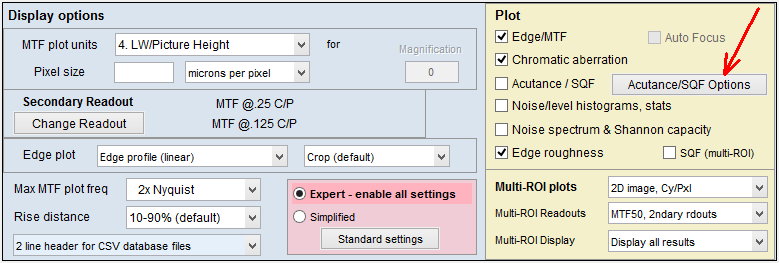Imatest SFR input dialog, showing SQF/Acutance

Clicking on to the right of the SQF checkbox opens this dialog box for setting SQF options. Most of the time you’ll want to leave them unchanged (except for Maximum print height, which doesn’t affect the calculations) at their default values, which you can always restore by pressing or .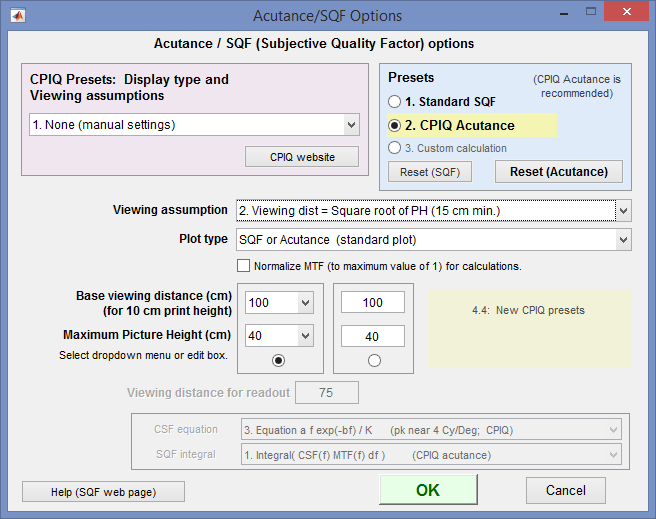Acutance settings

The 2. CPIQ Acutance preset is recommended. 1. Standard SQF is mostly of historical interest. 3. Custom calculation accesses some experimental calculations (not generally recommended).

Viewing assumption allows four choices.

1. Fixed viewing distance, which can be selected in the Base viewing distance… box.
2. Viewing distance Square root of PH (15 cm min.) (the default setting) assumes that viewing distance is proportional to the square root of the picture height, ($$d = (text{base distance})timessqrt{frac{PH}{10}}$$), with a minimum of 15 cm. This is the recommended setting, appropriate for typical gallery viewing. We tend to look at large prints at greater distances than small prints. With this assumption, if you viewed a 4x6 inch print at 12 inches (a number often found in the literature), you would view a 16x24 inch print at 24 inches.
3. Viewing distance = Cube root of PH (15 cm min.) assumes that viewing distance is proportional to the cube root of the picture height, ($$d = (text{base distance}) timessqrt{frac{PH}{10}}$$). With this assumption, if you viewed a 4x6 inch print at 12 inches (a number often found in the literature), you would view a 32x48 inch print at 24 inches. This seems to be a little close, but may be appropriate in some situations.
4. Fixed print height; Distance from 1 cm to max. This option is different from the others. Maximum viewing distance (cm) and Print height (cm) are entered instead of Base viewing distance and Maximum print height. SQF is plotted for viewing distances from 1 cm to the maximum for the fixed print height.

Of course Viewing distance is a broad average: we often move in and out when we critically examine a print. But some assumption must be made for the SQF/Acutance calculation to proceed. We believe that choice 2, Viewing distance proportional the square root of the picture height, best represents the typical impression of sharpness for a range of prints. But many developers require an analysis at a fixed viewing distance or display size.

Base distance Defined according to the Viewing assumption setting. It should be left at its default value of 30 cm (12 inches) unless there is good reason to change it. 34 centimeters has also been used for measuring perceived quality in 10 cm high (4x6 inch) prints.

For 1. Fixed viewing distance, base distance is the viewing distance in cm.

For Viewing distance = 2. Square root or 3. Cube root (of picture height), base distance is the viewing distance for 10 cm (4 inch) high prints.

Maximum print height is the maximum to plot. (Note that the height setting assumes landscape orientation: wider than tall.) It has no effect on the calculations. The default is 40 cm (16 inches), which is about as large as prints from consumer digital cameras get. Picture heights of 60 cm (24 inches) and larger are of interest to users of professional-quality digital SLRs. 20 cm is a stretch for camera phone images.

or resets settings to their default values. (It doesn’t affect Maximum print height, which has no effect on the calculations.) We recommend keeping all settings at their default values, unless there is good reason to change them. This will help ensure standardized measurements and minimize confusion.

Viewing distance for readout (cm) (for 4. Fixed print height) or Image height for readout (cm) (for 1. Fixed viewing distance) specifies a single viewing condition (distance and image height) for a readout on the SQF/Acutance plot.

## Acutance and SQF equations

Following the convention elsewhere in the Imatest site, we put the math in green boxes, which can be skipped by non-technical readers. The Acutance equation, which is recommended by the Camera Phone Image Quality (CPIQ) group is shown first. SQF is primarily of historical interest.

 The Acutance equation $$displaystyle text{Acutance} = frac{int_0^infty SFR_L (v) CSF(v) dv}{ int_0^infty CSF(v) dv}$$ where $$displaystyle CSF_L(v) = frac{av^c e^{-bv}}{K}$$ where a = 75, b = 0.2, c = 0.8, and K = 34.05, and ν is angular frequency with units of cycles/degree. Note that this value of CSF peaks around 4 cycles/degree, much lower than the CSF used for SQF. But SQF uses $$d(log f) = df / f$$, which compensates so that the final numbers are rather close. The SQF equation The gist of this box is that Granger presented the full equation for calculating SQF in 1972, but he used a simplified approximation for his calculations. Although the exact equation is strongly recommended, Imatest can use the simplified approximation where needed for comparing new and old calculations. In reviewing older publications, you should determine which calculation was used. The exact equation for SQF implied by Granger is, $$displaystyle SQF = K int_0^infty CSF(f) MTF(f) d(log f) = K int_0^infty frac{CSF(f) MTF(f)}{f} df$$  where CSF( f ) is the contrast sensitivity function of the human eye, discussed below; CSF( f ) is close to zero for f > 60 cycles/degree), MTF( f ) is the Modulation Transfer function (equivalent to Spatial Frequency Response) of the optical system: the primary output of Imatest SFR, log (often written ln) is the natural logarithm, i.e., loge. $$d(log f) = frac{df}{f}$$. $$displaystyle K = frac{100%}{int CSF(f) d(log f)} = frac{ 100%}{int frac{CSF(f)}{f} df}$$  is the normalization constant: SQF would equal 100% for MTF( f ) with a constant value of 1. The Nyquist frequency is the upper frequency limit for the integral; energy above Nyquist does not contribute to image quality. Since Granger had limited access to sophisticated computers (the average personal computer today has about as much power as the entire Pentagon had in 1972), he used an an approximation for his calculations assuming that CSF( f ) is roughly constant from 3 to 12 cycles/degree. $$displaystyle SQF = K int MTF(f) d(log f) = K int frac{MTF(f)}{f} df$$ for 3*cpd < f < 12*cpd; 0 otherwise; $$displaystyle K = frac{100%}{int d (log f)} = frac{100%}{ int frac{df}{f}}$$ for 3 cpd < f < 12 cpd is the normalization constant. $$displaystyle K = frac{100%}{log (12) – log (3)} = 72.1348%$$  Although Imatest offers the option of using this approximation (as a check on older calculations), the exact equation is recommended. The integration limits used by Granger and Cupery were 10 and 40 cycles/mm in the retina of the eye, which translate to 3 and 6 cycles/degree when the eye’s focal length (FL = 17 mm) is considered. f (cycles/degree) = f (cycles/mm) (π FL) / 180. To calculate SQF it is necessary to relate spatial frequency in cycles/degree, which is used for the eye’s response CSF( f ), to spatial frequency in cycles/pixel, which is calculated by Imatest SFR. $$displaystyle f(text{cycles/degree}) = frac{pi: n_{PH}: d: f(text{cycles/pixel})}{180: PH}$$ where nPH is the number of vertical pixels (along the Picture Height, assuming landscape orientation), d is the viewing distance PH is the picture height in units of distance (centimeters is used in Imatest displays). Note that nPH / PH is the actual value of pixels per distance. The “pixels per inch” value of image files rarely corresponds to real prints. Noisy images In images with long transitions (10-90% risetime r1090 over 2 pixels) and high noise, increasing the noise can increase the SQF, unless we take preventative steps. What we do is to recognize that most signal energy is at spatial frequencies below 1/r1090. At frequencies over 1/r1090 we do not allow MTF to increase: MTF( fn ) = min( MTF( fn-1 ), MTF( fn ) ). This has little or no effect on the MTF due to the edge, but prevents noise spikes from unduly increasing SQF.

## Contrast sensitivity function (CSF)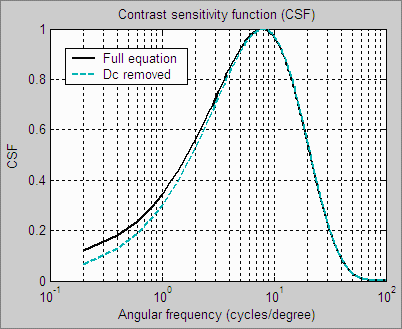Contrast sensitivity function

The human eye’s contrast sensitivity function (CSF) is limited by the eye’s optical system and cone density at high spatial (or angular) frequencies and by signal processing in the retina (neuronal interactions; lateral inhibition) at low frequencies. Various studies place the peak response at bright light levels (typical of print viewing conditions) between 6 and 8 cycles per degree (around 4 for the CSF equation used for acutance). We have chosen a formula, described below, that peaks just below 8 cycles/degree.

You may learn something about your own eye’s CSF by viewing the chart below at various distances and observing where the pattern appears to vanish. Chart contrast is proportional to (y/h)2, for image height h. To my eyes (which underwent Lasik refractive surgery in 1998 to correct for 10 diopter nearsightedness) it appears that the peak in the curve on the right should be considerably broader. But this isn’t quite the case because of the eye’s nonlinear response to contrast. Although the chart below appears to decrease in contrast linearly from top to bottom, the middle of the chart has 1/4 the contrast as the top.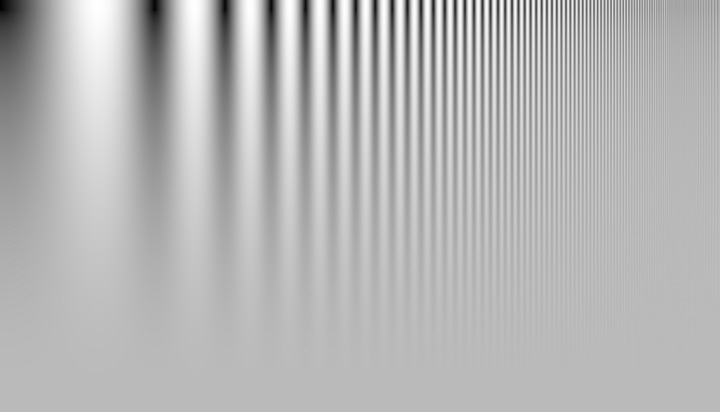Log frequency-Contrast chart, created by Test Charts.
The Log F-Contrast chart is very similar to the Campbell-Robson CSF (Contrast Sensitivity Function) chart,
which was first published in 1968. The (vertical) contrast variation is quite different for the two charts.

 For SQF, we have chosen a formula that is relatively simple, recent, and provides a good fit to data. The source is J. L. Mannos, D. J. Sakrison, “The Effects of a Visual Fidelity Criterion on the Encoding of Images”, IEEE Transactions on Information Theory, pp. 525-535, Vol. 20, No 4, (1974), cited on this page of Kresimir Matkovic’s 1998 PhD thesis. $$displaystyle CSF (f) = 2.6 ( 0.0192 + 0.114 f) e^{-{(0.114 f)}^{1.1}}$$ The 2.6 multiplier drops out of SQF when the normalization constant K is applied. This equation can be simplified somewhat. $$CSF(f) = (0.0192 + 0.114 f) e^{-0.1254f}$$  The preferred SQF equation, $$SQF = K int CSF(f) MTF(f) d(log f) = K int frac{CSF(f) MTF(f)}{f} df$$, blows up when the dc-term (0.0192) is present. Fortunately, as the above plot shows, removing it makes very little difference. The formula used to calculate SQF in the preferred equation is CSF( f ) = 0.114 f exp(-0.1254 f ).

 More geek stuff (additional equations that have been deprecated) Some additional equations were included in the SQF options for experimental study.They have been deprecated because acutance has become a standard. $$SQF = K int_0^{infty}! CSF(f) MTF(f) , mathrm{d} log f ,, = K int_0^{infty}! (CSF(f) MTF(f))/f , mathrm{d} f$$ where  $$K = frac{100%}{int CSF (f) df}$$ or $$K = frac{100%}{log (int CSF(f) df)}$$ is the normalization constant. log (often written ln) is the natural logarithm, i.e., loge. These equations were developed to address concerns about d(log f ) = d f /f , which becomes very large at low spatial frequencies. Integrals containing this term blow up if CSF( f ) contains a dc (constant) term. Fortunately, as noted above, removing the dc term from CSF makes very little difference and works quite well, so we only keep these equations for experimental purposes. Granger used d(log f ) because he noticed that a constant percentage change in MTF corresponded to a just noticeable difference, i.e., the eye responds logarithmically. Equation 1 is linear. Equation 2 is logarithmic, but presents some scaling problems: What to do about log(1) = 0? Should we use log10 (comparable to density measurements), log2 (comparable to exposure value or f-stop measurements), or loge (in accordance with Boulder, Colorado community standards, where only organic, natural logarithms are employed)? We’ve chosen not to pursue these equations.